# How to Use Excel Formulas Multiply - Part 2

## Introduction

In part 1 of this article we learned the basics of using Excel formulas to multiply. In this article we'll build upon this knowledge. We will again site examples and put this method into practice by performing some exercises which will demonstrate the process step by step.

Let us understand what is a PRODUCT function before advancing to the method itself.

### PRODUCT Function Syntax:

=PRODUCT(number1, [number2], ...)

PRODUCT function "multiplies all the numbers given as arguments", it belongs to the category Math & Trig on Excel groups under the Formulas tab.

### Multiply using PRODUCT function

It is a lot better to use this function to multiply on cells, ranges or numbers. This is much easier to use and more sustainable as the size of your spreadsheets grow.

Rather than putting the asterisk (*) in between the numbers we want to get the product of, we can put the function PRODUCT after the equal sign (=) in our formula, then just click the cell or range of our cells we want to perform the multiplication on.

#### Example 1

Let us site an example where excel formulas multiply using PRODUCT function can be used:

For Numbers:

The Formula is =PRODUCT(1,2,3)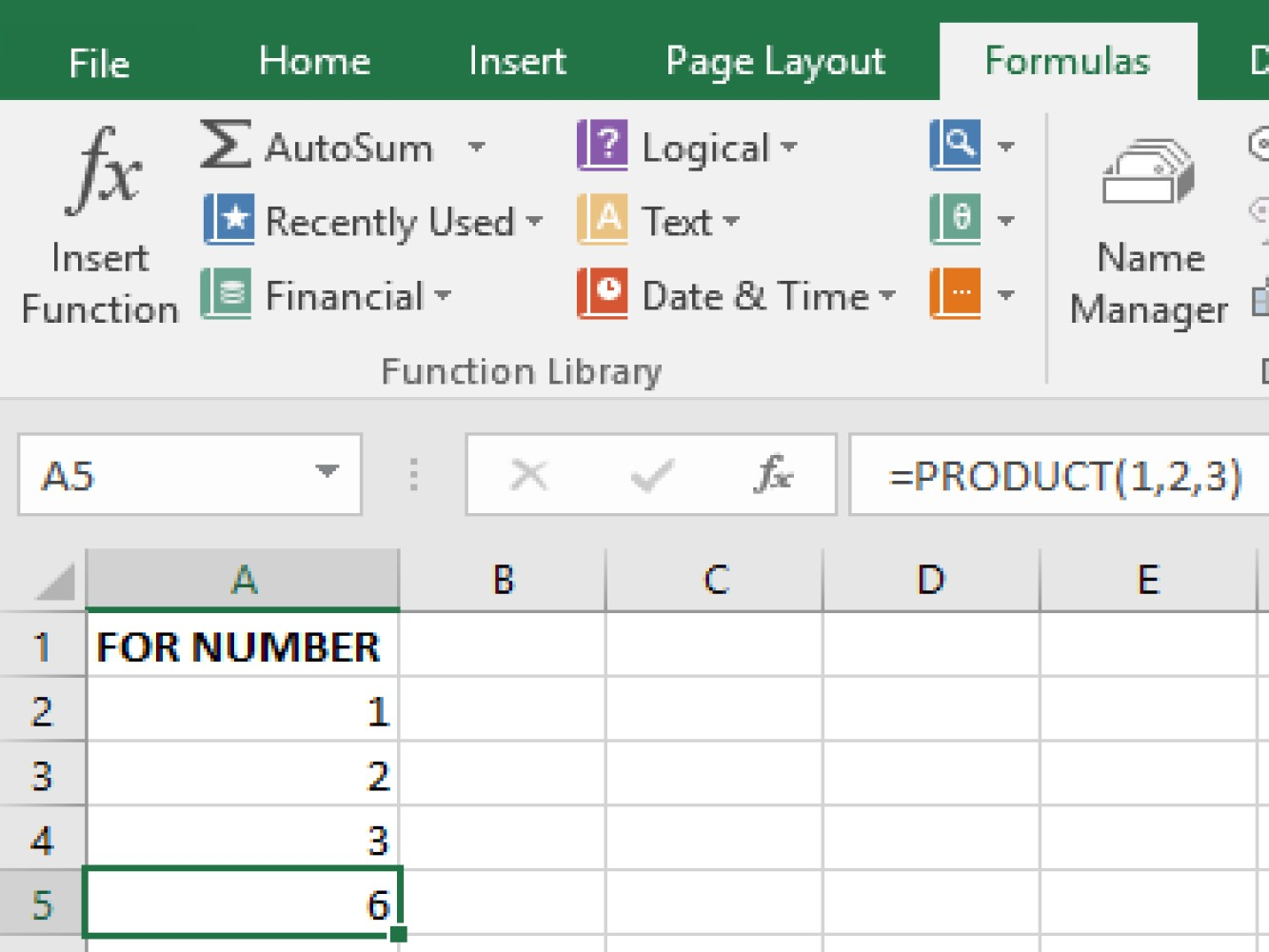A result of 6 came out of our example. Note that the PRODUCT() function in this example did not depend at all on the other cells in the spreadsheet because the numbers were hardcoded into the formula. If you deleted all the other cells, the cell A5 with the formula in it would still behave the same because it is not dependent on other cells.

#### Example 2 - Using Cell References

To do the same calculation you could use the following formula =PRODUCT(A1:A3). Notice that unlike in Example 1, this formula does depend on other cells. It depends on the cells A1 through A3 which is the specified range passed into the PRODUCT() function.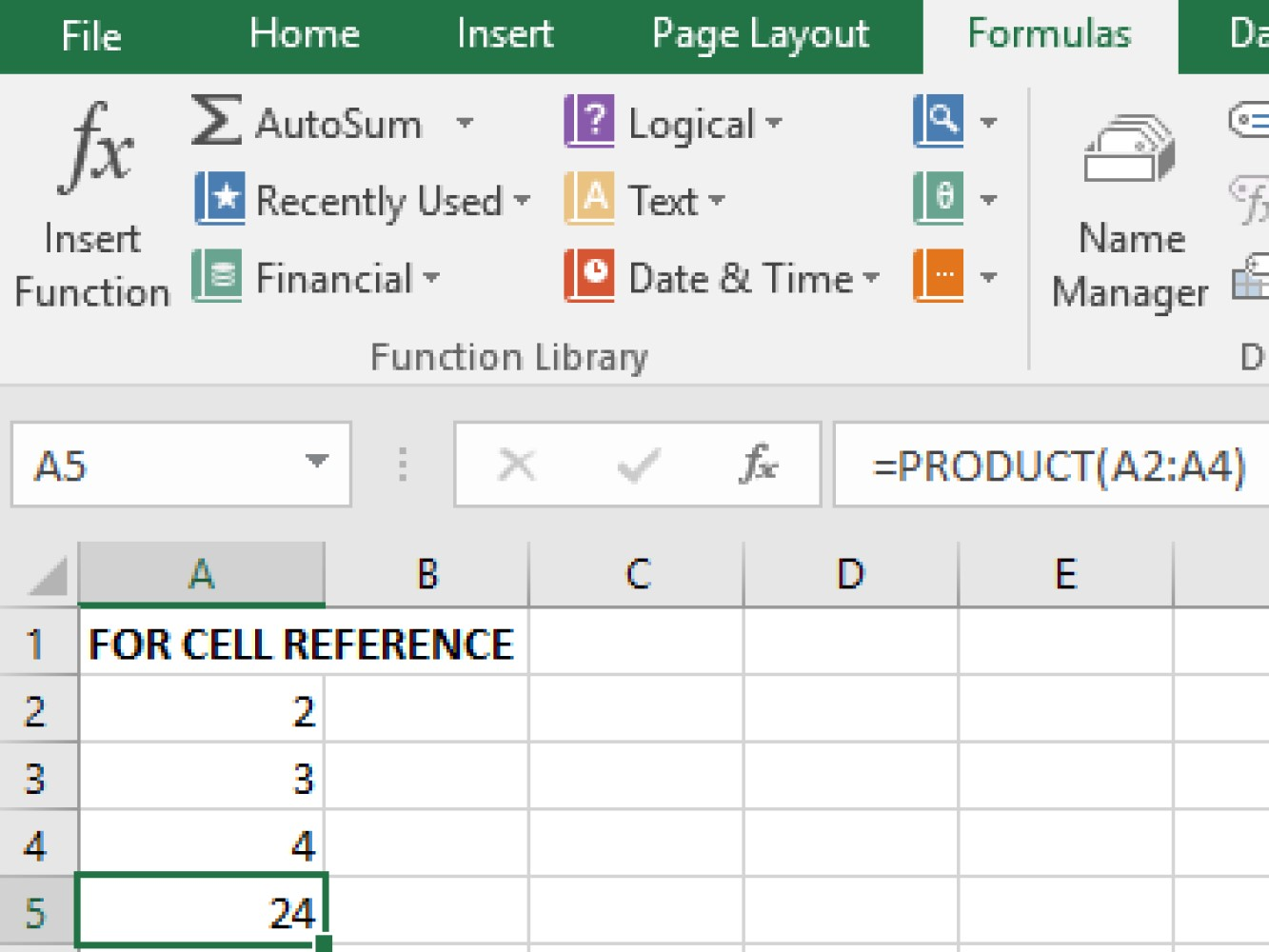A result of 24 came out of our example.

#### Example 3 - Dragging multiply formulas in rows

Once you have a formula for a given range, you can drag that formula out so that it applies to other similar ranges ( other columns in this case. )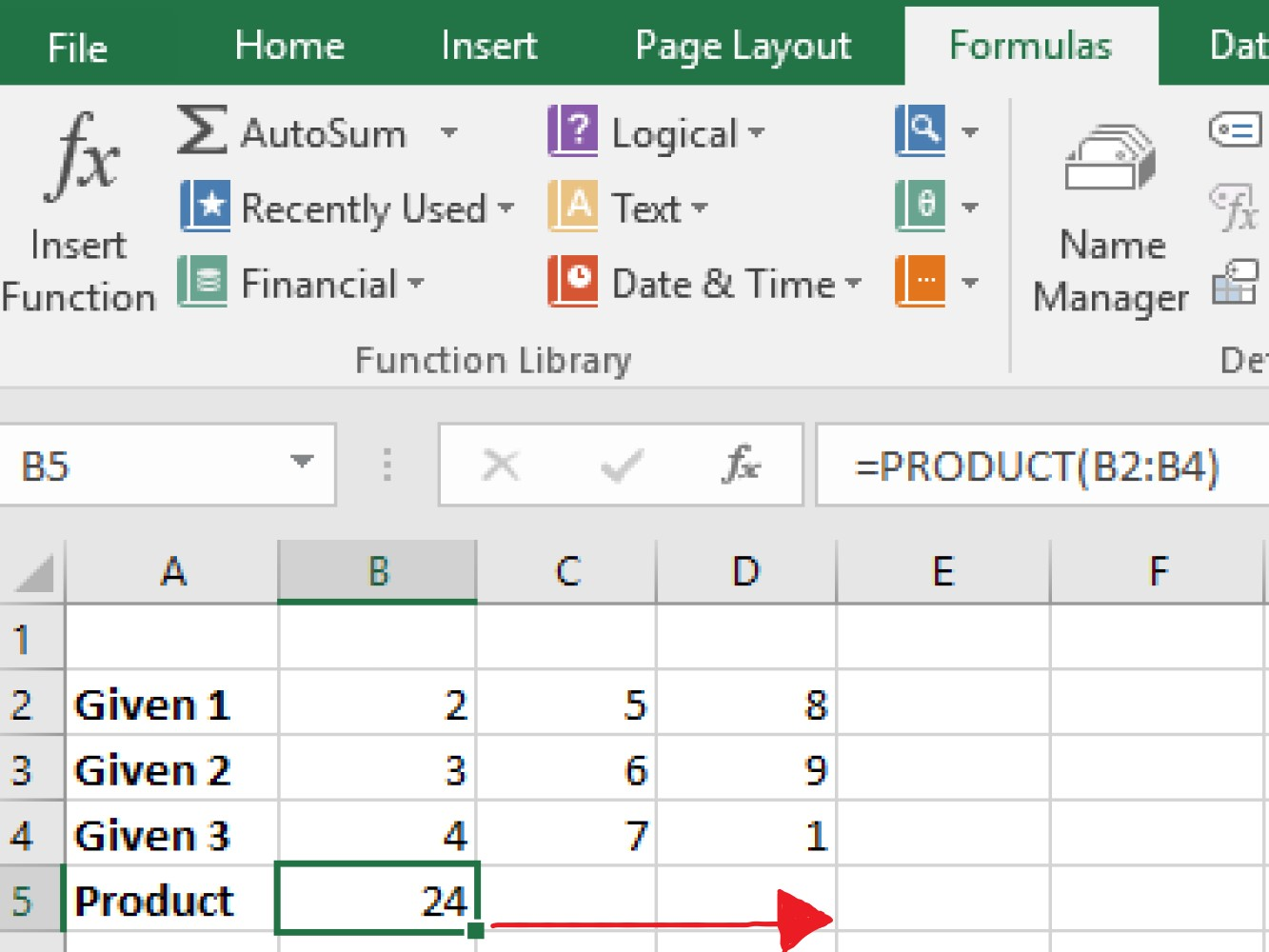Now below is an example of applying a formula to multiple rows by dragging.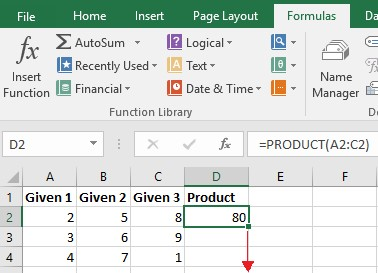TIPS

Another way to copy the formula is by highlighting the range where you wanna copy the formula with and press keyboard shortcut Ctrl+ D regardless if its vertical for columns and horizontal for rows.

### Multiply using Percentage

The percentage can also be easily multiplied by using an excel spreadsheet.

The formula in multiplying the percentage by number: =10*10%

The formula in multiplying the percentage by cell: =A2*10%

Or you can transform a percentage on a decimal number example: =10*0.1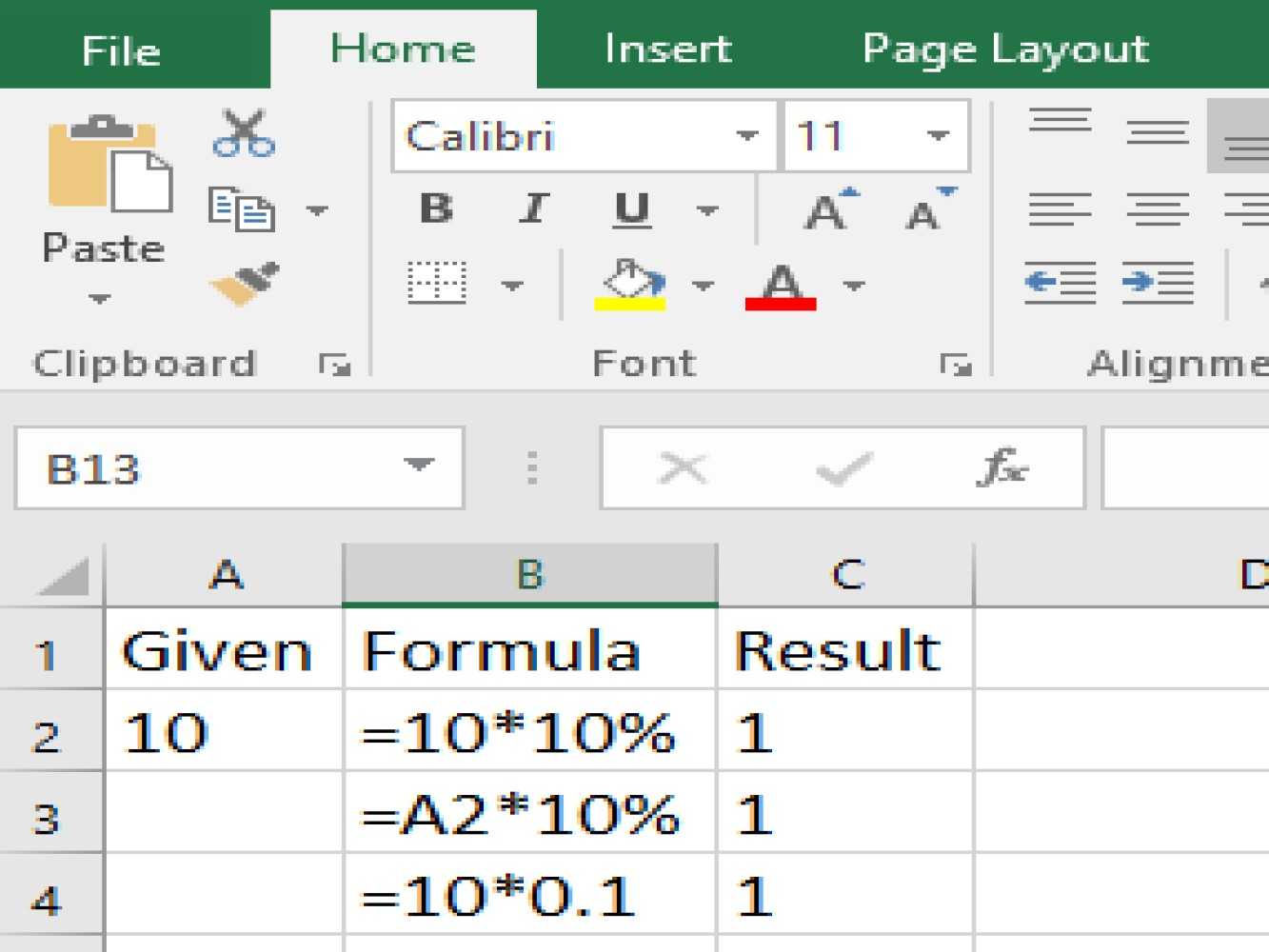#### Formulas for Increasing and Decreasing % value:

Formula to increase an amount by % :

= Given * ( 1 + % )

For example, =A1*(1+10%)

Formula to decrease an amount by % :

= Given* ( 1 - % )

For example, =A1 * (1-10%)

TAKE NOTE

When you use a cell reference in a formula the answer or value of the product can be changed by simply changing the given numbers we wish to multiply unless we make the given an absolute reference where it won't change even if we copy it to a different part of our spreadsheet

## Conclusion

This concludes this multi-part article on the different methods of how to use excel formulas multiply. We hope you feel more comfortable with the options to do multiplication and can put them in practice.

Here's the links for each part of the article:An innovative marketing agency specializing in marketing tactics designed to produce positive results.